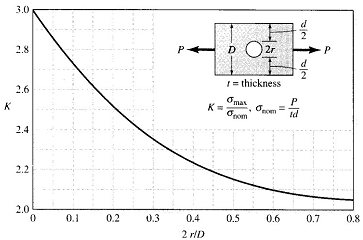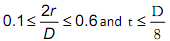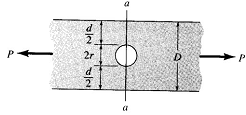### Construct a suitable finite element model

Assignment Help Mechanical Engineering
##### Reference no: EM13103004

The figure below shows the geometrical stress concentration factor, K for a flat bar loaded in uniaxial tension. The bar has a uniform rectangular cross-section.Working with values within the rangeyour task is to:

1. Construct a suitable finite element model and compare the FEA predictions with the theoretical data.

2. Produce a graph (within ANSYS) to show the stress distribution normal to the cross-section along line a-a shown here.Verified Expert

#### Determine the molecular weight of a poly

Determine the molecular weight of a poly(ethylene-propylene) with a degree of polymerization of 25,000 given that 20% of the mers (by number) are ethylene and 80% are propyl

#### Sketch the streakline of the smoke

Sketch the streakline of the smoke, the pathline of the first particles leaving the chimney, and a few streamlines, assuming that the wind blows parallel to the ground in a

#### What is the cost of gasoline model that makes this option

what is the cost of gasoline (dollars per gallon) that makes this option affordable when the buyer 's interest rate is 10% per year? The car will be driven 100,000 miles uni

#### Find the amount of return air and outdoor air in cfm

A building has a calculated cooling load of 24 tons, of which 6 tons is latent load. The space is to be maintained at 72 F db and 50% relative humidity. 15% by volume of the a

#### Determine the rate of heat transfer from the air

Air enters the evaporator at 14.7 psia and 90 F with a volumetric flow rate of 200 ft3/min. Refrigerant 134a at 20 psia with a quality of 30 % enters the evaporator at a rate

#### Calculate the maximum addendum possible for the rack

A pinion with a pitch radius of 38.0 mm chives a rack. The pressure angle is 2°. Calculate the maximum addendum possible for the rack without having involute inter-ference o

#### Non-reacting gaseous mixture with molar analysis

A non-reacting gaseous mixture with molar analysis of 74.3% N2, 4.3% O2, 7.1% CO2, and 14.3% H2O (g) enters a counter-flow heat exchanger at 800?F and 1 atm with a volumetric

#### Find the rectangle of largest area

Use the method of Lagrange multipliers to find the rectangle of largest area, with sides parallel to the axes, that can be inscribed in the ellipse (x / a)2 +

### Write a Review